##### Pre-Calculus: 1001 Practice Problems For Dummies (+ Free Online Practice)Using the periodicity identities comes in handy when you need to prove an equality that includes the expression (x + 2pi) or the addition (or subtraction) of the period. For example, to prove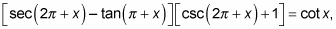1. Replace all trig functions with the appropriate periodicity identity.

You're left with (sec x – tan x)(csc x + 1).

2. Simplify the new expression.

For this example, the best place to start is to FOIL: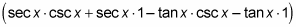Now convert all terms to sines and cosines to get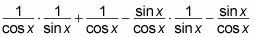Then find a common denominator and add the fractions: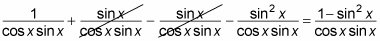3. Apply any other applicable identities.

You have a Pythagorean identity in the form of 1 – sin2 x, so replace it with cos2 x. Cancel one of the cosines in the numerator (because it's squared) with the cosine in the denominator to get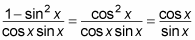Finally, this equation simplifies to cot x = cot x.